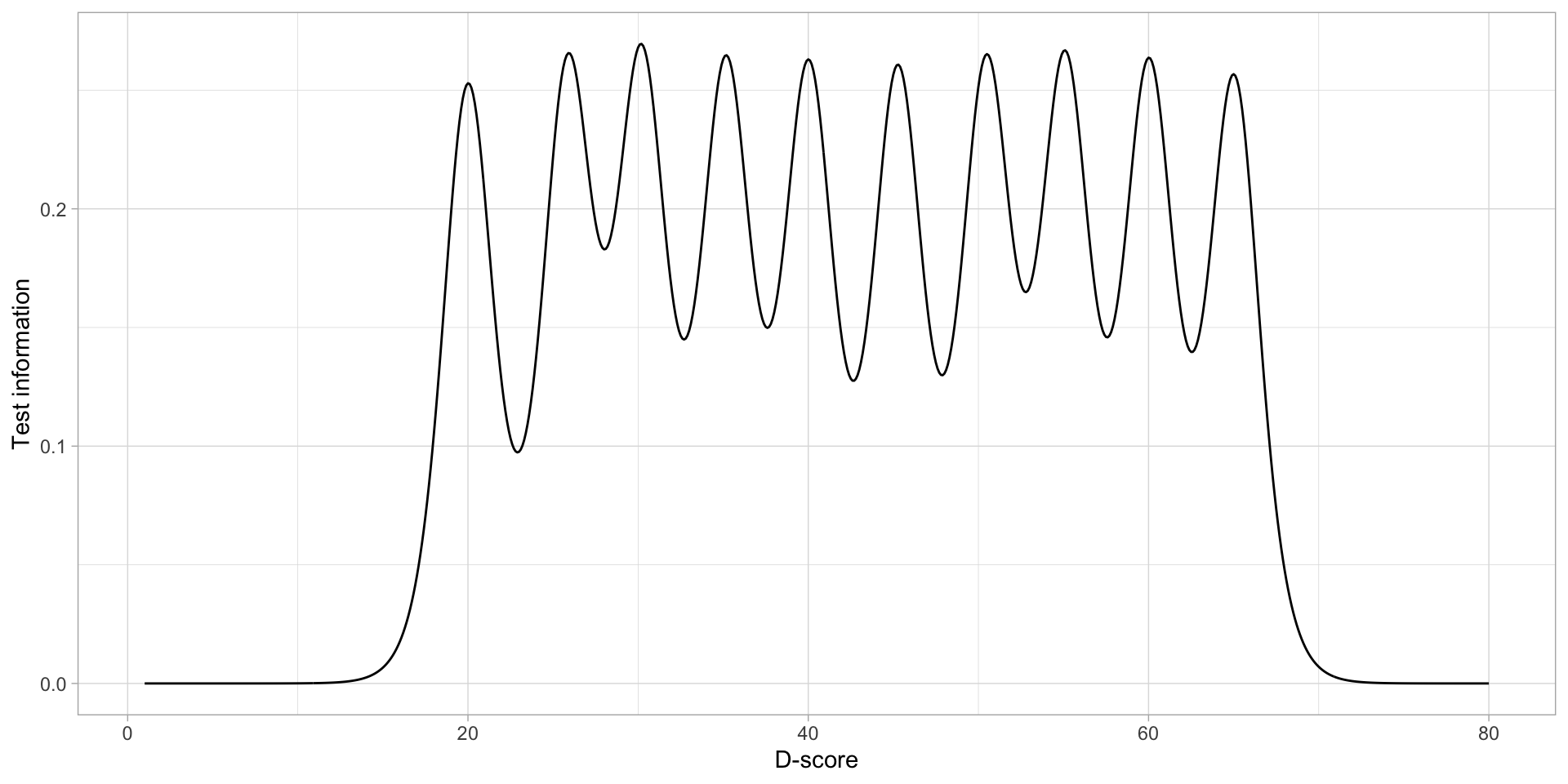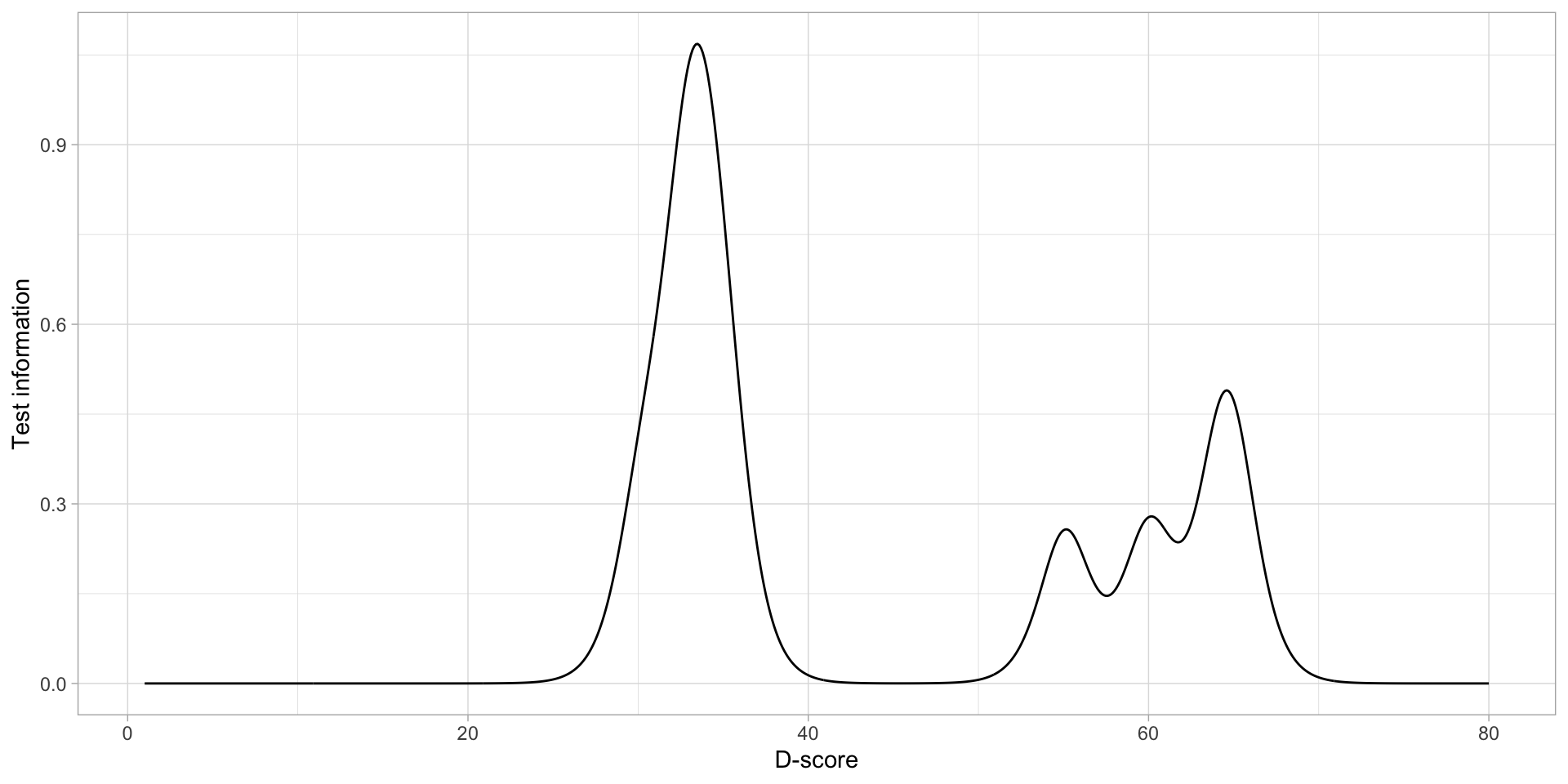## 3.3 Test information by ability

The sum of the item information at each point of the $$D$$-score scale holds the test information for the ability. Figure 3.5 shows the test information for the first set of items. This shows a uniform level of information over the $$D$$-score scale.

The test information for the second set of items is extremely high at 33$$D$$. Between a $$D$$ of 40 and 50 there is no information in the test and at 65$$D$$ there is another peak in information. This shows that the test information for the second set of items is not evenly distributed over the $$D$$-score range.Figure 3.5: (fig:testinfo1)Figure 3.6: (fig:testinfo2)